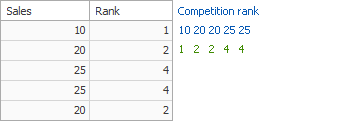20.2
20.1
19.2
19.1
The page you are viewing does not exist in version 19.1. This link will take you to the root page.
18.2
The page you are viewing does not exist in version 18.2. This link will take you to the root page.
18.1
The page you are viewing does not exist in version 18.1. This link will take you to the root page.
17.2
The page you are viewing does not exist in version 17.2. This link will take you to the root page.

# Window Calculations Overview

Window calculations use window functions to calculate data across a set of window rows related to the current row. Partitioning criteria are applied to rows to arrange them in windows.

##### NOTE

Window calculations are available for the Optimized calculation engine. Use the PivotGridOptionsData.DataProcessingEngine property to enable the Optimized engine.

The following calculation types are supported:

## Running Total

Allows you to calculate a cumulative total for a set of measure values.

Expression

``````RunningSum(Sum([Sales]))
``````

Field Data Binding

Binding Partitioning Parameters
RunningTotalBindingBase ColumnValue SummaryType = Sum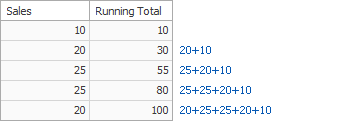## Moving Calculation

Allows you to apply a moving calculation, which uses neighboring values to calculate a total. Note that neighboring values are specified using offsets from the currently processed value.

Expression

``````WindowSum(Sum([Sales]), -1, 1)
``````

Field Data Binding

Binding Partitioning Parameters
MovingCalculationBindingBase ColumnValue SummaryType = Sum, PreviousValuesCount = 1, NextValuesCount = 1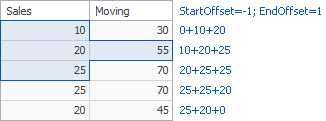## Difference

Allows you to compute differences between measure values.

Expression

``````Sum([Sales]) - Lookup(Sum([Sales]), -1)
``````

Field Data Binding

Binding Partitioning Parameters
DifferenceBindingBase ColumnValue Target = Previous, DifferenceType = Absolute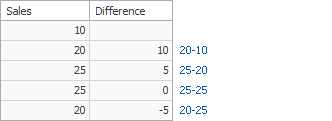## Percent of Total

Allows you to calculate a contribution of individual measure values to a total.

Expression

``````Sum([Sales]) / Total(Sum([Sales]))
``````

Field Data Binding

Binding Partitioning Parameters
PercentOfTotalBindingBase ColumnValue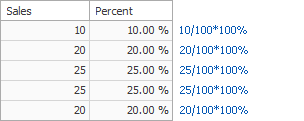## Rank

Allows you to rank values of the specified measure.

Expression

``````RankCompetition(Sum([Sales]), 'asc')
``````

Field Data Binding

Binding Partitioning Parameters
RankBindingBase ColumnValue RankType = Competition Order = Ascending